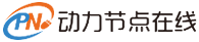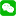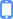#微信登录微信登录与注册手机号登录

# 算法时间复杂度

04-07 22:24 641浏览

• 大字
• 中字
• 小字

# 定义

O()来提现算法时间复杂度的记法，称为大O记法

# 推导大O阶方法

• 用常数1取代运行时间中的所有加法常数
• 在修改后的运行次数函数中，只保留最高阶项
• 如果最高阶项数存在而且不是1，则去除与这个项相乘的常数
• 得到的最后结果就是大O阶

``````int n = 100, sum = 0;
for(int i = 0; i < n; i++)
{
sum += i;
}``````

``````int i, j, n = 100;
for (i = 0; i < n; i++)
{
for (j = 0; j < n; j++)
{
printf("hello");
}
}``````

``````int i, j, n = 100;
for (i = 0; i < n; i++)
{
for (j = i; j < n; j++)
{
printf("hello");
}
}``````

0人推荐

0条评论

Java初学者学习方法

Java中模拟高并发的方法

Java程序员必备的一些流程图

## 举报

0/150